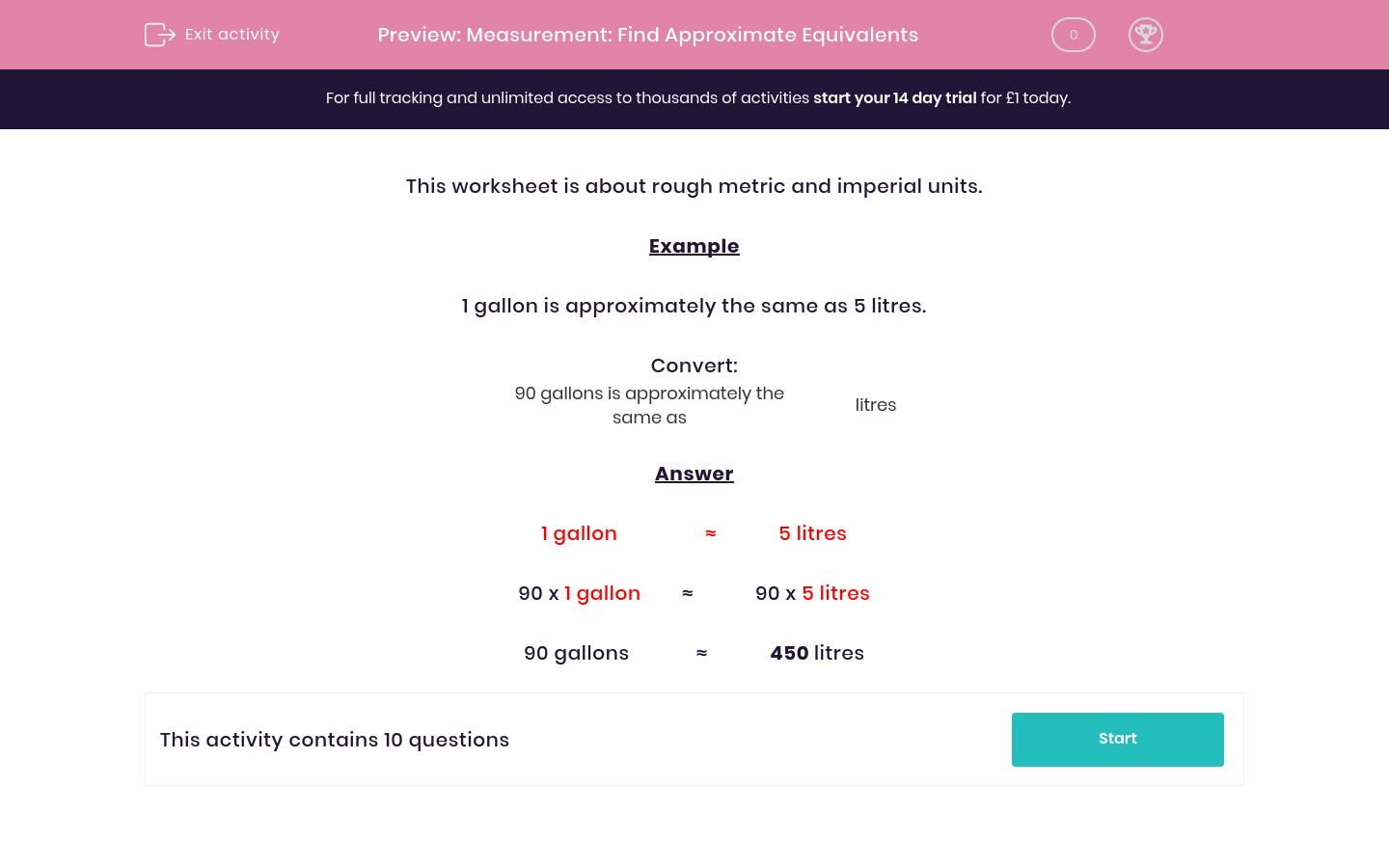# Measurement: Find Approximate Equivalents

In this worksheet, students find approximate imperial and metric equivalents by scaling measurements up.Key stage:  KS 2

Curriculum topic:   Maths and Numerical Reasoning

Curriculum subtopic:   Metric & Imperial

Difficulty level:### QUESTION 1 of 10

This worksheet is about rough metric and imperial units.

Example

1 gallon is approximately the same as 5 litres.

Convert:

90 gallons is approximately the same as  litres

1 gallon                 ≈            5 litres

90 x 1 gallon        ≈            90 x 5 litres

90 gallons             ≈            450 litres

1 gallon is approximately the same as 5 litres.

Complete:

10 gallons is approximately the same as    litres

1 gallon is approximately the same as 5 litres.

Complete:

15 gallons is approximately the same as    litres

1 gallon is approximately the same as 5 litres.

Complete:

50 gallons is approximately the same as    litres

1 gallon is approximately the same as 5 litres.

Complete:

20 gallons is approximately the same as    litres

1 mile is approximately the same as 1.5 km.

Complete:

2 miles is approximately the same as  km

1 mile is approximately the same as 1.5 km.

Complete:

10 miles is approximately the same as  km

1 mile is approximately the same as 1.5 km.

Complete:

8 miles is approximately the same as  km

1 mile is approximately the same as 1.5 km.

Complete:

3 miles is approximately the same as  km

1 gallon is approximately the same as 5 litres.

Complete:

80 gallons is approximately the same as    litres

1 gallon is approximately the same as 5 litres.

Complete:

70 gallons is approximately the same as    litres
• Question 1

1 gallon is approximately the same as 5 litres.

Complete:

10 gallons is approximately the same as    litres
50
• Question 2

1 gallon is approximately the same as 5 litres.

Complete:

15 gallons is approximately the same as    litres
75
• Question 3

1 gallon is approximately the same as 5 litres.

Complete:

50 gallons is approximately the same as    litres
250
• Question 4

1 gallon is approximately the same as 5 litres.

Complete:

20 gallons is approximately the same as    litres
100
• Question 5

1 mile is approximately the same as 1.5 km.

Complete:

2 miles is approximately the same as  km
3
• Question 6

1 mile is approximately the same as 1.5 km.

Complete:

10 miles is approximately the same as  km
15
• Question 7

1 mile is approximately the same as 1.5 km.

Complete:

8 miles is approximately the same as  km
12
• Question 8

1 mile is approximately the same as 1.5 km.

Complete:

3 miles is approximately the same as  km
4.5
• Question 9

1 gallon is approximately the same as 5 litres.

Complete:

80 gallons is approximately the same as    litres
400
• Question 10

1 gallon is approximately the same as 5 litres.

Complete:

70 gallons is approximately the same as    litres
350
---- OR ----

Sign up for a £1 trial so you can track and measure your child's progress on this activity.

### What is EdPlace?

We're your National Curriculum aligned online education content provider helping each child succeed in English, maths and science from year 1 to GCSE. With an EdPlace account you’ll be able to track and measure progress, helping each child achieve their best. We build confidence and attainment by personalising each child’s learning at a level that suits them.

Get started